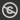Science, Maths & Technology
Free course

# Rings and polynomials## Course reviews

This free course contains an introduction to rings and polynomials. We see that polynomial rings have many properties in common with the integers; for example, we can define a division algorithm, and this enables us to develop the analogue of the highest common factor for two polynomials. Section 1 explores the abstract definitions of a ring and a field. Sections 2 and 3 define polynomial rings where the coefficients of the polynomials are elements from a given field. Section 3 develops results concerning the divisibility of polynomials.

## Course learning outcomes

After studying this course, you should be able to:

• recall and be able to use the axioms that define a ring, and know the basic properties of rings arising from these axioms
• know how to add and multiply polynomials over arbitrary fields, and be able to use this to define polynomial rings
• understand the statement and proof of the Division Algorithm for polynomials, and be able to apply polynomial long division in the ring Q[x]
• understand the meaning of the highest common factor of two polynomials, the proof of existence of the hcf, the meaning of 'coprime' in the context of polynomials over fields, and be able to apply the Euclidean Algorithm to compute the hcf of two polynomials f and g in Q[x], and find polynomials a, b such that hcf(f, g) = af + bg
• understand the meaning of the least common multiple of two polynomials, the proof of its uniqueness, and be able to compute lcms in the polynomial ring Q[x].

First Published: 09/03/2018

Updated: 23/05/2018

Skip Rate and Review